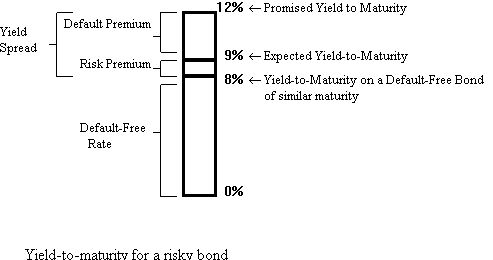# Fixed Income Portfolio ManagementP.V. Viswanath, 2000

## Interest Rate Risk

### Reinvestment Risk

Coupon bonds are subject to Reinvestment Risk. If the bondholder has a horizon longer than the first coupon payment date of the bond, at least some of the coupons must be reinvested. If the level of interest rates is low, the coupons must be reinvested at a low rate; on the other hand, if the level is high, the investor can get a high rate.

All bonds are subject to Capital Gain Risk, if the maturity of the bond differs from the bondholder's horizon.

### Capital Gain Risk:

Consider a 2 year, 10% coupon bond with a \$1000 face value, with a yield of 8.8%. The price of the bond equals 50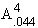+ 1000/(1.09)2 = 50 x 3.596 + 841.68 = 1021.46, whereis the annuity factor representing the present value of \$1 paid at the end of 4 periods, with a periodic interest rate of 4.4%.

Suppose the market interest rate drops to 7.8%. The market price is now given by 50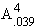+ 1000/(1.039)4 = 1040.02.

As the bond yield drops, the bond price rises, and vice-versa.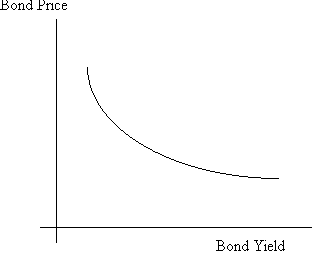### Interplay of Capital Gain Risk and Reinvestment Risk

An investor with a horizon of 1 year buys the 2 year, 10% coupon bond.

Scenario 1: The interest rate drops to 7.8%, soon after the bond is purchased, and stays there. The bond price rises immediately to 1040.02. After six months, the bond price rises to 1040.02 (1.039) = 1080.58. At this time, a coupon of \$50 is paid, whereupon the price of the bond drops by an equal amount to 1030.58. The bond increases in value at the end of the year to 1030.58 (1.039) = 1070.77, while the \$50 coupon is reinvested at an annual yield of 7.8% and grows to 50 (1.039) = 51.95, for a total of 1122.72.

Scenario 2: The interest rate rises to 8.2%, and stays there. The price immediately drops to 50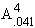+ 1000/(1.041)4 = 1032.59. However, the bond and coupons thereafter rise at the rate of 4.1% every six month, culiminating in a value of 1119.00 at the end of the investment horizon.

What we see is that if interest rates drop and stay there, there is an immediate appreciation in the value of the portfolio, but the portfolio then grows at a slower rate; on the other hand, if interest rates rise and stay there, there is a capital loss, but the portfolio then appreciates more rapidly. Hence there is some investment horizon, such that investors with that horizon, will not care if interest rates drop or rise (as long as the changes are small). This horizon is shown in the figure below as D. We will see below that the value of D depends on the characteristics of the bond portfolio; specifically, we will see that D is simply the duration of the bond portfolio.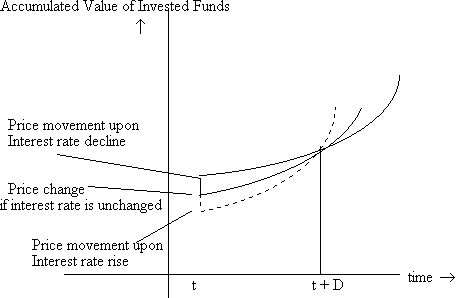## Measurement of Interest Rate Risk

### The Macaulay Duration Measure Defined

A stock's risk is measured by its beta, which looks at the stock's sensitivity to market movements. The same thing could be done, in principle, with bonds. However, there are two further issues: one, interest rates are the main source of risk for bonds; two, obtaining return data on bonds to measure a market beta is difficult because a) bonds are often not traded; and b) the maturity of a bond changes with time, and hence its identity. Consequently, bond price risk is, most often, measured in terms of the bond's Interest Rate Sensitivity.

Bond pricing formula where y is the yield and Ci, the cash flow in period i: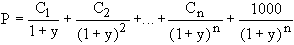Assuming that the yield curve moves parallel to the initial yield curve, we can differentiate with respect to the yield, y, to obtain:, where D is the Macaulay duration and is defined as: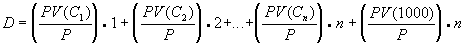, where

present values are computed using the yield to maturity, y.

Consider a 2 year, 10% coupon bond selling at par. In this case, the case flows are as follows:

 Time 0.5 1 1.5 2 Cash flow 50 50 50 1050 PV/Price 0.0476 0.04535 0.04319 0.8638 (PV/Price)xTime 0.0238 0.04535 0.064785 1.7277
In this case, the yield is simply 5% for six months, and the duration can be computed simply as above. This gives us a duration of 1.8616 years.

Modified Duration = Macaulay Duration/(1+y)

Hence, Modified Duration x P = delta(P)/delta(y)

### Properties of the Macaulay Duration measure:

• The duration of a zero-coupon bond equals its time to maturity.
• Holding maturity constant, a bond's duration is higher when the coupon rate is lower.
• Holding the coupon rate constant, a bond's duration generally increases with its time to maturity. Duration always increases with maturity for bonds selling at par or at a premium to par.
• Holding other factors constant, the duration of a coupon bond is higher when the bond's yield to maturity is lower.
• The duration of a level perpetuity is (1+y)/y.
• For a bond with a constant coupon, duration is computed as:where y is the yield per payment period, c is the coupon rate per payment period, and n is the number of periods.
• For bonds selling at par, duration is measured by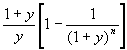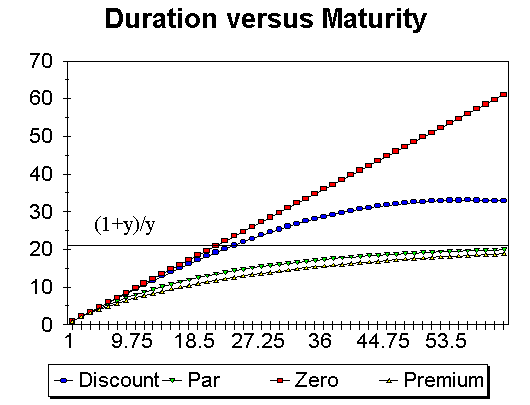### Approximating price changes using duration:

From the formula for duration, we can write dP = [- D P/(1+y)]dy,

where dy is the yield change, and dP is the price change.

Example:

We computed the duration of a 2 year, 10% coupon bond selling at par as 1.8616 years or 3.7232 half-years. For this bond, if the yield changes from 5% per 6 months to 4.5%, the price change can be estimated to be [-3.7232 x 100/1.05] 0.005 = \$1.77, whereas the actual price change is given by 100 - [5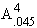+ 100/(1.045)4], or \$1.79.

This formula only works for small yield changes, because the price of a bond as a function of yield is nonlinear. In other words, the duration of a bond changes as the yield changes. The price approximation is improved if we can take into account this nonlinearity. This is what is known as convexity.

Convexity is defined as (d2P/dy2)(1/P), and can be computed as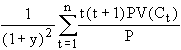.

For bonds with a constant coupon of \$C per period, there is a closed-form solution for the second derivative: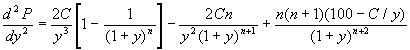Note: Convexity in years = Convexity in m periods per year/m2, while Duration in years = Duration in m periods per year/m.

### Approximating price change using duration and convexity:

The estimated percentage change in price due to duration and convexity is:

dP/P = -D* (dy) + 0.5 (Convexity) (dy)2

For a 6%, 25-year bond selling to yield 9%, the modified duration works out to 10.62 years, while the convexity is 182.92 (in years). The estimated percentage price change, for a yield change from 9% to 11% is -10.62 (0.02) +0.5 (182.92) (0.02)2 = -21.24 + 3.66% = -17.58%, while the actual change is -18.03%.

If the yield decreases by 200 basis points, instead, then the estimated price change is +21.24% due to duration, and +3.66 due to convexity, or 24.90%; the actual price change is 25.46%. The estimated price change is no longer symmetric about the current yield, because the price function has curvature.

Example: Compute the convexity for an 5 yr., 8% bond selling at par.
We use the formula.

 Period Cash Flow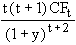1 4 7.1119709 2 4 20.515301 3 4 39.452501 4 4 63.225162 5 4 91.190138 6 4 122.75595 7 4 157.37943 8 4 194.56248 9 4 233.84914 10 104 7145.3902 Sum 8075.4323
Second derivative (d2P/dy2)= 8075.43

convexity in half years (d2P/dy2)(1/P) = 8075.43/100 = 80.7543

Convexity in years = 80.7543/4 = 20.1885

Dollar convexity = Price x convexity = 100 x 20.1885 = 2,018.85

### Convexity and Bond PricingBond B has greater convexity than Bond A. Whether the yield rises or falls, Bond B will have a higher price. Hence, while it may happen at times that two bonds of different convexities will be priced similarly, as in the figure above, normally convexity will be priced. Ceteris paribus, bond B will command a higher price than bond A.

How much should the market want investors to pay up for convexity? If investors expect that yields will not change much, then there is not much of an advantage to higher convexity, while if yields are expected to change a lot, convexity will be highly valued. In general, investors with higher than average volatility expectations will be willing to pay for buy convexity (buy the higher convexity bond and sell the lower convexity bond), while investors with lower than average volatility expectations would be willing to sell convexity.

### Non-parallel yield curve changes

Duration as a measure of interest rate risk assumes that the yield curve moves in parallel. What happens if the yield curve does not move in parallel?

Over the 1930-1979 period, the following was found to be true: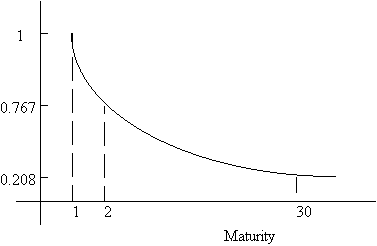Average Change in YTM as a multiple of the change in YTM for 1 year bonds.

That is, long term rates are less volatile than short term rates. Immunization must therefore take this into account. More complex formulas have been computed allowing for particular kinds of non-parallel shifts in the yield curve, but this added complexity does not seem to have paid off in more effective immunization. Work currently under way models the term structure as composed of two (or more) stochastic factors. It is then possible to write the future value of any portfolio in terms of its exposure to the two (or more) factors. Under these circumstances, immunization would imply equating the sensitivities of asset and liability portfolios to the stochastic factors.

### Effects of non-parallel yield curve shifts on immunized portfolios

Consider the following two portfolios:

Portfolio I, referred to as the 'bullet' portfolio consists of Bond C.

Portfolio II, called the barbell portfolio, consists of 50.2% of bond A and 49.8% of bond B.

 Bond Coupon Maturity (yrs.) Price plus Accrued Yield Dollar Duration Dollar Convexity A 8.50 5 100 8.50 4.00544 19.8164 B 9.50 20 100 9.50 8.88151 124.1702 C 9.25 10 100 9.25 6.43409 55.4506
The duration of both portfolios is equal to 6.43409.

The convexity of the two portfolios differs: The bullet portfolio has a dollar convexity of 55.4506, while that of the barbell portfolio is 71.7846.  (Note that consistent with the higher convexity of the barbell portfolio, it has a higher price, i.e. a lower yield. This yield can be computed as a weighted average of the yields of the two bonds making up the portfolio, and it works out to 8.998%.)

Given the similar durations of the two portolios, the bullet and barbell portfolios will perform similarly for small changes in yield (if the yield curve changes in parallel). However, for larger changes, since the barbell portfolio has larger convexity, it will do relatively better than the bullet portfolio.

However, we cannot make similar claims if the yield curve changes in a non-parallel fashion. When the yield curve flattens, the barbell portfolio dominates the bullet portfolio, while for a steeper yield curve, the barbell is better, for large drops or large rises in the yield, but worse otherwise.

###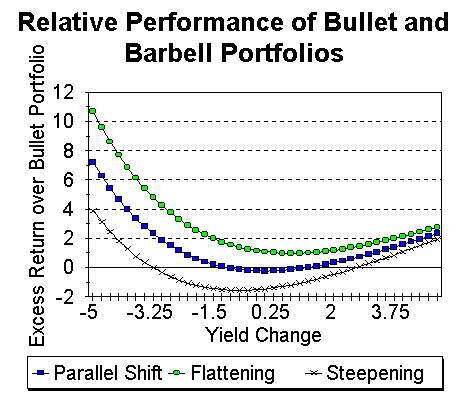Yield Change Parallel Shift Flattening Steepening -5 7.19 10.68 3.88 -4 3.99 6.88 1.27 -3 1.88 4.25 -0.37 -2 0.59 2.55 -1.25 -1 -0.07 1.54 -1.57 0 -0.25 1.06 -1.48 1 -0.09 0.98 -1.09 2 0.31 1.18 -0.49 3 0.89 1.58 0.24 4 1.57 2.12 1.06 5 2.31 2.75 1.92

• Flattening: Yield Change bond A = Yield change bond C + 25 basis points
• Yield Change bond B = Yield change bond C - 25 basis points
• Steepening: Yield Change bond A = Yield change bond C - 25 basis points
• Yield Change bond B = Yield change bond C + 25 basis points
In addition to flattening and steepening, yield curves can also become less humped (positive butterfly change) or more humped (negative butterfly change).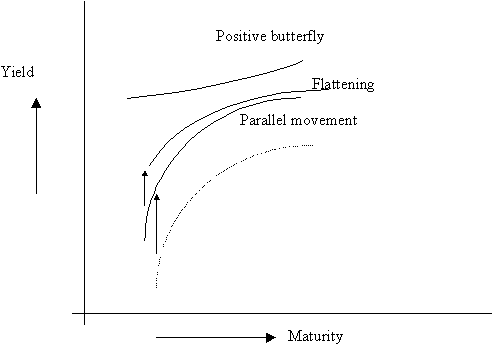Butterfly changes account for about 3.4% of Treasury returns, while parallel shifts and twists account for 91.6%, with 5% unknown. (Frank Jones, "Yield Curve Strategies," Journal of Fixed Income, Sept. 1991, pp. 43-48.)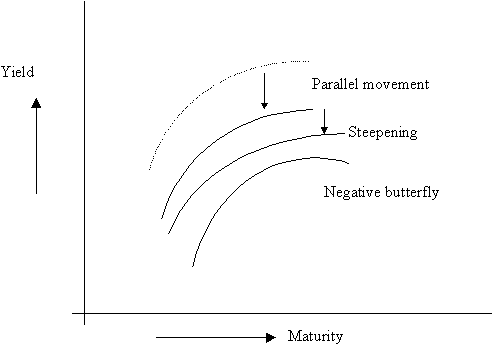Empirically, an upward shift and flattening of the yield curve is accompanied by a positive butterfly movement (less humped), while a downward shift and a steepening of the yield curve is correlated with a negative butterfly movement.

The following strategies have been shown to work will under the following different scenarios.

 Yield Decreases Yield Increases SteeperYield Curve Intermediate Maturity  Bullet Short Maturity  Bullet Flatter Yield Curve Long Maturity Barbell (around long maturity) Short or Long Maturity  (greater flattening relative to upward yield shift favors the long maturity)

A positive butterfly movement implies that yields on intermediate maturity bonds drop in price and hence a movement towards an intermediate maturity is warranted.

# Bond Portfolio Management

Bond Portfolios can be managed actively or passively. Passive bond managers take bond prices as given and seek only to adjust the risk of their portfolios. Active managers believe that they can spot bond mispricing and/or predict future trends in bond prices.

### Passive Bond Portfolio Management

Passive bond management can be accomplished in two ways:
• attempt to replicate the performance of a given bond index;
• attempt to shield the overall exposure of his portfolio to interest rate fluctuations--this method is broadly called immunization.

#### Principles of Bond Indexing

The idea is to create a portfolio that mirrors the composition of an index that measures the broad market (e.g. the S&P 500 Index is used for equity index funds).

Bond Indexes:

There are three major bond indexes of the broad bond market: the Salomon Brothers Broad Investment Grade (BIG), the Lehman Brothers Aggregate Index, and the Merrill Lynch Domestic Master Index. All three indexes inlcude bonds with maturities greater than one year; as the maturity of a bond falls below a year, it is dropped from the index.

Considerations in choosing an index:

• the investor's risk tolerance
• the investor's objectives--is total return the objective, or is reduction of variability important?
• regulatory constraints. e.g. a regulated financial institution that is not permitted to invest in the high-yield sector of the corporate market should not select an index that includes that sector.
Difficulties in indexing:

There are several problems with tracking a bond index:

1. there are many securities (4000 to 5000) in a broad bond index,
2. many of these are thinly traded;
3. the composition of the index changes regularly, as they mature.
The performance of a portfolio tracking a given index is measured in terms of what is known as tracking error--the difference between the return on the index, and that on the tracking portfolio. Tracking error has two main components: error due to transactions costs, and error due to differences in composition between the index and the tracking portfolio. A tracking portfolio that mimics the index exactly will have high tracking error from the first component and none on the second component. The objective is to balance both kinds of error.

#### Bond Indexation Techniques

Three different techniques are used in bond indexing:
• Cellular
• Optimization and
• Variance Minimization.
The Cellular approach is a stratified sampling approach. Bonds are identified according to its characteristics, such as: a) duration; b) coupon rate;

c) maturity; d) market sectors (Treasury, corporate, mortgage-backed, etc.); e) credit rating; f) call factors; and g) sinking fund features.

The objective is then to select from all of the issues in the index, one or more issues in each cell that can be used to represent the entire cell. The total dollar amount invested in each cell will depend upon the share of that cell in the entire universe of securities represented in the index. The number of cells used will depend on the dollar amount of the portfolio to be indexed. A portfolio with a small dollar value will have fewer cells, so as not to increase tracking error due to transactions costs.

The Optimization approach is also a cellular one; however, the choice of securities from each cell is made with a view to achieving some other objective, such as e.g. maximizing yield to maturity, maximizing convexity, etc;  Mathematical programming is used to achieve this goal.

The Variance Minimization approach uses historical data to estimate the variance of the tracking error for each issue in the index, and then use that to minimize the total variance of the tracking error. The tracking error variance of a given security is obtained by estimating the price function for that security as a function of its cash flows, duration, and other sector characteristics. Quadratic programming is then used to find the optimal index portfolio in terms of minimized tracking error. Since it is difficult to get enough data on each security, this approach is very difficult.

### Immunization

#### Net Worth Immunization

This approach is adopted by institutions that would like to reduce variation in their net market worth, e.g. banks. There may be regulatory implications regarding infusions of fresh capital if net worth falls below certain limits. This objective can be broadly achieved by ensuring that the duration of the asset portfolio equal the duration of the liability portfolio if the present value of the assets equals the present value of the liabilities. If this second condition is not satisfied, then equating durations will not necessarily work, because:

Net Worth = PV(A) - PV(L) = [-DAPV(A) + DLPV(L)]y/(1+y), which is not equal to zero, where Di , i = A, L stands for the duration of the assets and the liabilities respectively.  Hence equating durations will work only if PV(A) = PV(L). In general the condition that must be satisfied is:

DAPV(A) = DLPV(L)

If we define immunization as a strategy that ensures that the terminal value is not smaller than the initial value, then we can write the necessary conditions as:

Duration(assets) = Duration(liabilities)

PV(Assets) greater than or equal to PV(Liabilities)

There are additional complexities if there are multiple liabilities, as is usually the case. This is dealt with later. In addition, Gap Managers may also be interested in keeping the ratio of Net Worth to Assets or some other measure immunized with respect to interest rate changes.

#### Target Date Immunization

This approach is adopted by institutions that are concerned, not just that the net worth remain relatively constant, but that each promised payment (liability) be made on the appropriate date. The two objectives are very similar, and in properly functioning markets, should be the same. Obviously, if each liability is immunized, all liabilities together are also immunized.  However, if net worth is immunized, each liability by itself might not be immunized, and it would be necessary to depend upon higher asset revaluations overall to make up shortfalls in the funding for particular liabilities.

It is worthwhile at this point to revisit the twin effects of a yield change.Consider a portfolio with a value of V at time t*-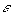(the instant before t*). If the yield on the portfolio is y, the portfolio value will increase at the rate y. Let D be the duration of the portfolio. At time t* + D, the value of the portfolio is V(1+y)D. Now suppose the yield increases at time t*, so that the portfolio value drops to V1. However, because of the higher yield, the portfolio value increases at the higher rate y1. In how many periods, will the portfolio value reach V(1+y)D? It can be shown that this happens in exactly D periods.

That is, V(1+y)D = V1(1+y1)D

In other words, as explained earlier in the webnote, the duration of a portfolio is exactly the amount of time that it takes for the change in value due to a yield increase to be made up by the increased reinvestment rate. This also makes it clear that if an insurer desires to have a certain amount of money, say \$10,000 available for payouts in k years, and the discounted present value of those \$10,000 is available today (i.e. the liability if fully funded), then those funds must be invested in a portfolio with a duration of k years. In this way, the portfolio is immunized against changes in value for small changes in the yield. Alternatively, the duration of the asset portfolio must match that of the liability portfolio.

We have below a graph of portfolio value as a function of yield, assuming that there is one yield change immediately, and none thereafter: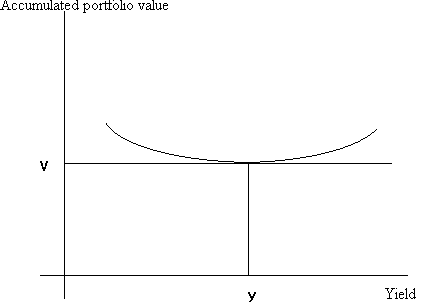Note that the accumulate portfolio value remains at V for very small changes in y. However, for larger changes in y, the portfolio value increases, both for yield increases and for yield decreases. (This is due to bond convexity.)

Example:

Consider an insurance company with a single liability of 17,183,033 in exactly 5.5 years. Suppose the company purchases an 8-year, 10.125% bond selling to yield 12.5%. Assume coupons can be reinvested at this rate.

In order to fund the liability fully, the company would need to purchase bonds worth 17,183,033/(1.0625)11 = 8,820,062.20. The price of an 8-year 10.125% bond selling to yield 12.5% can be computed to be= 9.9346(5.0625) + 51.33 = 88.20262; hence the face value of the bonds purchased is 8,820,062.20/88.20262 = \$10 m. Suppose the yield changes to 12%. In this case, the value of the portfolio would rise to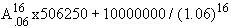= 5,116,109.5 + 3,936,462.8 = 9,052,572. This would grow to 9052572(1.06)11 = 17184484 at the end of 5.5 years for a gain of \$1451. It can be confirmed that the value of the portfolio would be greater than the target 17183033 for any yield change at all.

Note that the duration of the 8-year 10.125% bond selling at a price of 88.20 is exactly 5.5 years.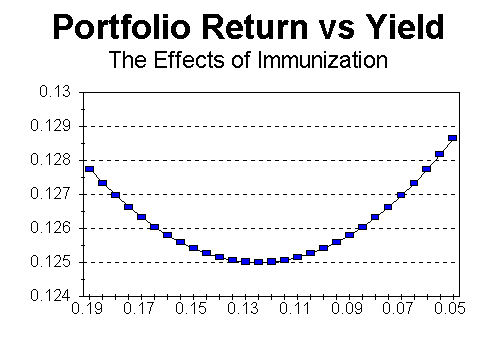#### Immunization with multiple liabilities

When there is more than one liability, it is not enough to equate liability and asset durations, even if the liabilities are fully funded, and even if only parallel yield curve changes are assumed. An additional condition must be satisfied: the cash flows of the individual portfolio assets must be more widely dispersed than those of the liabilities. With a single cashflow liability, the convexity of the liability cannot be greater than the convexity of the asset portfolio immunizing the liability, but when liabilities with multiple cashflows are to be considered, convexity must be explicitly taken into account to ensure that the convexity of the liability does not exceed the convexity of the immunizing assets. This is the reason for the dispersion condition. If the dispersion condition is not satisfied, then for large interest rate changes, increases in the value of the liability may not be offset by increases in the value of the asset.

The way this additional condition works can be illustrated graphically as follows: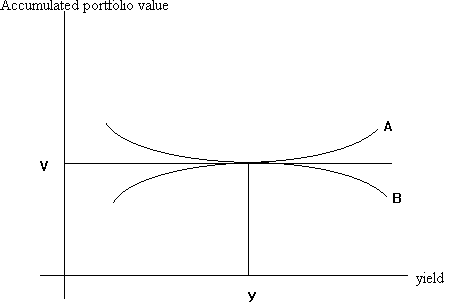Both portfolios A and B satisfy the duration condition: the value of both portfolios remain constant for very small changes in yield. However, for larger changes in yield, portfolio B will lose value, and will not be immunized.

Mathematically, dispersion is given by the following formula: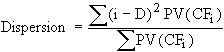, where

CFi is the cash flow at time i, and D is the duration of the bond portfolio.

Rebalancing in an immunization strategy

The duration of a single period liability will change at the rate of 1 per year, while that of the asset portfolio will not necessarily change so smoothly. This also applies to multi-period liabilities. (Durations will decrease smoothly until a coupon payment is made.) Hence, it will be necessary to rebalance the asset portfolio to maintain the equality of durations, even if yields do not change.

Cash flow matching and dedication

Cash flow matching implies constructing an asset portfolio that has cash flows that are equal in magnitude and opposite in sign to the obligation. In a multiperiod context, cash flow matching is called dedication.

#### Choosing from alternative immunizing portfolios

In general, there will exist more than one portfolio that satisfies the immunization conditions. If so, the manager will want to choose the best portfolio. One strategy is to pick the immunizing portfolio with the greatest yield. This permits immunization at the least cost.

The following graph shows how duration is affected when bonds are combined into portfolios.Climbing The Immunization Curve

Consider a given liability portfolio. It's duration depends on the rate used to discount the portfolio's cash flows. The 'immunization curve' relates duration to yield. Suppose there are four assets, A, B, D, and E. We need to find a portfolio of these four assets with the same duration as our liability. IF we discount the liability portfolio using the yield on the portfolio of assets, we can see that there are several such portfolios--a, b, and e. Since portfolio b has the highest yield, we would prefer that portfolio, so that we can minimize the total investment required for immunization.

Of course, this procedure makes the strong assumption that the yield curve is flat at the yield level of the asset portfolio; hence one must be careful not to rely too heavily on this technique.### Active Bond Management

One can distinguish between two aspects of active bond portfolio management:
• interest rate forecasting, and
• identification of relative mispricing within the fixed-income market.
Homer and Liebowitz characterize active bond portfolio management in terms of four types of bond swaps:
• the substitution swap: exchanging one type of bond for another which is nearly identical. This is motivated by a belief that there is a temporary mispricing.
• the intermarket spread sway: this is motivated by a belief that the spread between two sectors of the bond market, say, between corporates and Treasuries is too large or too small. Again, the spread is expected to move to a more 'appropriate' level soon.
• the rate anticipation swap: if investors believe that interest rates will fall, they will switch into bonds of higher duration; conversely, if interest rates are expected to rise, they will switch into bonds of lower duration, to be positioned to take advantage of the higher rates without suffering a capital loss.
• the pure-yield pickup swap: one way of doing this is by riding the yield curve. In general, the investor holds higher yield bonds in return for bearing higher credit risk or higher term structure risk.
Horizon analysis is a form of interest rate forecasting. This involves predicting the yield curve at different points in time in the future and using these predictions to estimate the total return from different bonds.

For example, if it is expected that the yield curve will remain fairly constant over time, then holding higher maturity bonds may be preferred to holding shorter maturity bonds. In this way, the bonds can be purchased at a high yield (low price) and then sold at a low yield (high price).

#### Contingent Immunization

A portfolio manager may have a minimum portfolio value in mind at a prespecified horizon point. He has two alternatives: one, to put the available money in a fully immunized portfolio that is guaranteed to attain the required minimum portfolio value at the horizon point. Alternatively, if he currently has a greater sum of money that is required in order to attain that minimum value, he may choose to invest it in a riskier portfolio, switching to the riskless strategy whenever his portfolio value drops to the level at which a riskless strategy will yield exactly the desired minimum portfolio value at the horizon point. Such a strategy may result in a switch to the riskless portfolio, at some time t*, as shown in the diagram below, or, if the manager's strategy is sufficiently successful, he may never have to switch to the riskless portfolio as in the second diagram.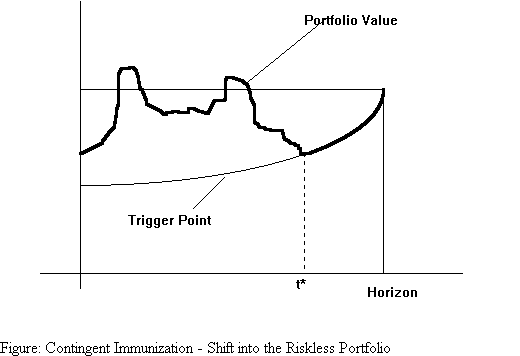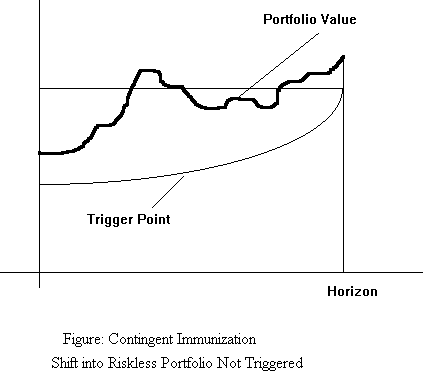### Credit Risk

#### Introduction

Bonds other than Treasury Bonds are subject to potential default of the bond issuer. This risk is termed credit risk. Therefore, the promised yield is the maximum possible yield to maturity of the bond, not necessarily the actual yield to maturity.

The expected yield to maturity can be computed as the weighted average of all possible yields, weighted by their probabilities.

To compensate investors for the possibility of bankruptcy, a corporate bond must offer a default premium. This default premium is a differential in promised yield between the corporate bond and its expected yield to maturity.

The default premium depends on two main factors:

• the probability of default
• the likely financial loss to the bondholder in the event of default.
If the probability of default, p, is constant from year to year, and the loss on a bond that defaults equals a proportion of the bond's market price in the previous year, then the default premium,

d = y -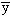(where y is the promised yield-to-maturity, andis the expected yield to maturity), can be given by: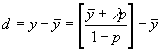Example:

Suppose Bond A has a promised yield-to-maturity of 12%, but owing to a high default risk, the expected yield is only 9%.

Assume that this bond has a 6% annual default probability and that it is estimated that if the bond does default, each bondholder willl receive an amount equal to 60% of the bond's market price a year earlier. What is the equilibrium default premium on this bond?

Using the above formula, the default premium of the bond should be

(.09 + 0.4x.06)/0.94 - .09 = 0.0313, or 3.13%. In other words, the promised yield should be 12.13%, which is relatively close to the 12%, assumed in the example.

The riskiness of individual bonds depends on various factors, such as:

• sales
• profitability (earnings before interest and taxes)
• cash reserves (retained earnings)
• liquidity (working capital)
• leverage (ratio of total debt to market value of equity)

#### Types of Ratings

Moody's Investors Service and Standard & Poor's Corporation provide ratings of the default risk of corporate bonds. Moody's designates the highest grade bonds by the letters Aaa (S&P uses AAA), followed by Aa (AA), A (A), Baa (BBB), Ba (BB) and B, and so on. These ratings are measures of relative risk, rather than of absolute risk. Consequently, the yields on bonds of different ratings move up and down together, but the spreads between them are not constant over time.

 Moody's S&P Investment Grade Bonds Aaa AAA Aa AA A A Baa BBB Speculative Grade Bonds Ba BB B B Caa CCC Ca CC C C